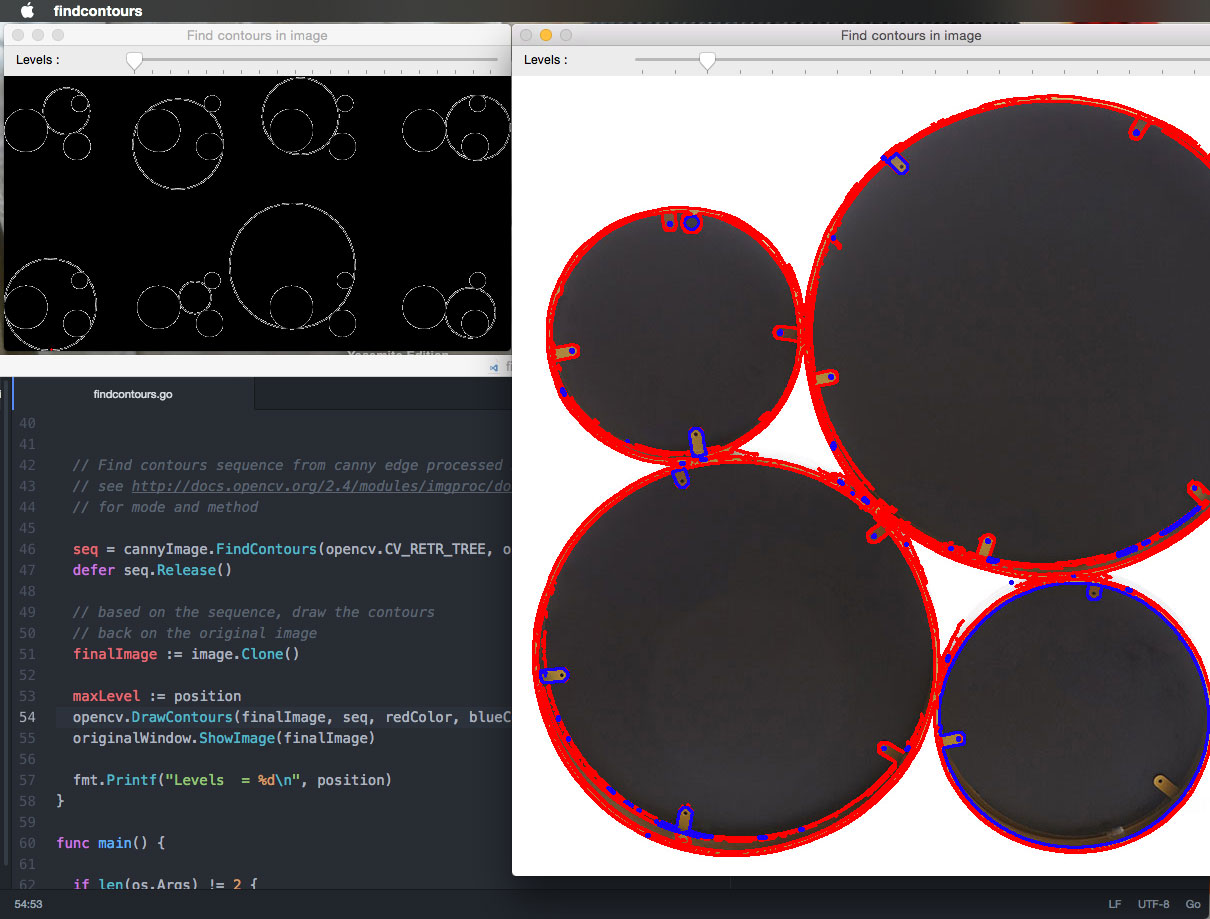# Golang : Find and draw contours with OpenCV example

Ability to detect shapes is often the most important steps in allowing a computer to make decision...such as deciding if the shape is a circle or rectangle. There are a couple of ways to detect shapes in an image. One of them is to find contours on the image.

Basically, contours are a set of points connected to each other to form an outline of an object(kinda like drawing along the dotted line in children coloring books)The Golang code example below illustrates how to extract contours with `FindContours()` function and draw contours back on the original image with `DrawContours()` function. Bear in mind that Golang implementation is slightly different from C/C++ or Python code examples found on OpenCV website, but the end result is the same.

Here you go!

`````` package main

import (
"fmt"
"github.com/lazywei/go-opencv/opencv"
"os"
)

var (
originalWindow = new(opencv.Window)
image = new(opencv.IplImage)
seq *opencv.Seq
redColor = opencv.NewScalar(0, 0, 255, 255) // (blue, green, red, alpha)
blackColor = opencv.NewScalar(0, 0, 0, 255) // (blue, green, red, alpha)
blueColor = opencv.NewScalar(255, 0, 0, 255) // (blue, green, red, alpha)
slider = 15
)

func trackBar(position int, param ...interface{}) {

width := image.Width()
height := image.Height()

// Convert to grayscale
gray := opencv.CreateImage(width, height, opencv.IPL_DEPTH_8U, 1)
defer gray.Release()

opencv.CvtColor(image, gray, opencv.CV_BGR2GRAY)

// for edge detection
cannyImage := opencv.CreateImage(width, height, opencv.IPL_DEPTH_8U, 1)
defer cannyImage.Release()

// Run the edge detector on grayscale
//opencv.Canny(gray, cannyImage, float64(position), float64(position)*2, 3)

// ** For better result, use 50 for the canny threshold instead of tying the value
// to the track bar position
opencv.Canny(gray, cannyImage, float64(50), float64(50)*2, 3)

// Find contours sequence from canny edge processed image
// see http://docs.opencv.org/2.4/modules/imgproc/doc/structural_analysis_and_shape_descriptors.html
// for mode and method

seq = cannyImage.FindContours(opencv.CV_RETR_TREE, opencv.CV_CHAIN_APPROX_NONE, opencv.Point{0, 0})
defer seq.Release()

// based on the sequence, draw the contours
// back on the original image
finalImage := image.Clone()

maxLevel := position
opencv.DrawContours(finalImage, seq, redColor, blueColor, maxLevel, 2, 8, opencv.Point{0, 0})
originalWindow.ShowImage(finalImage)

fmt.Printf("Levels  = %d\n", position)
}

func main() {

if len(os.Args) != 2 {
fmt.Printf("Usage : %s <image filename>\n", os.Args)
os.Exit(0)
}

imageFileName := os.Args

fmt.Println("Press ESC key to quit")

if image == nil {
}
defer image.Release()

originalWindow = opencv.NewWindow("Find contours in image")
defer originalWindow.Destroy()

originalWindow.CreateTrackbar("Levels : ", 0, slider, trackBar)

// initialize by drawing the top level contours(level = 0)
trackBar(0, 0)

for {
key := opencv.WaitKey(20)
if key == 27 {
os.Exit(0)
}
}

os.Exit(0)
}
``````

References:

https://socketloop.com/tutorials/golang-eroding-and-dilating-image-with-opencv-example

http://docs.opencv.org/3.2.0/d4/d73/tutorialpycontours_begin.html